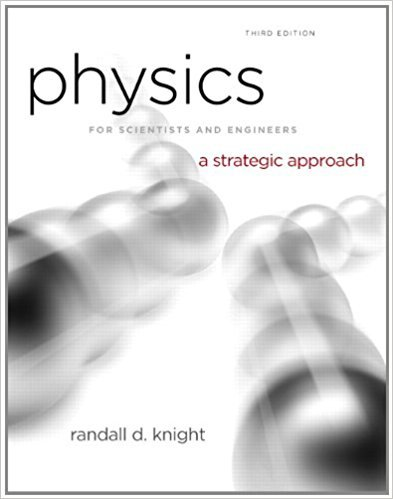×
×

# The four balls in FIGURE have been thrown straight up.ISBN: 9780321740908 69

## Solution for problem 9CQ Chapter 6

Physics for Scientists and Engineers: A Strategic Approach with Modern Physics | 3rd Edition

• Textbook Solutions
• 2901 Step-by-step solutions solved by professors and subject experts
• Get 24/7 help from StudySoup virtual teaching assistantsPhysics for Scientists and Engineers: A Strategic Approach with Modern Physics | 3rd Edition

4 5 1 290 Reviews
18
2
Problem 9CQ

The four balls in FIGURE have been thrown straight up. They have the same size, but different masses. Air resistance is negligible. Rank in order, from largest to smallest, the magnitude of the net force acting on each ball. Some may be equal. Give your answer in the form a > b = c > d and explain your ranking.

FIGUREStep-by-Step Solution:

Solution 9CQ

Step 1 of 1</p>

We have to rank in order, from largest to smallest, the magnitude of the net force acting on each ball.

The net force acting on the all the balls is the force due to gravity and it is given by the expression.Where,mass...

Step 2 of 3

Step 3 of 3

##### ISBN: 9780321740908

Since the solution to 9CQ from 6 chapter was answered, more than 5064 students have viewed the full step-by-step answer. The full step-by-step solution to problem: 9CQ from chapter: 6 was answered by , our top Physics solution expert on 08/30/17, 04:34AM. The answer to “The four balls in FIGURE have been thrown straight up. They have the same size, but different masses. Air resistance is negligible. Rank in order, from largest to smallest, the magnitude of the net force acting on each ball. Some may be equal. Give your answer in the form a > b = c > d and explain your ranking.FIGURE” is broken down into a number of easy to follow steps, and 60 words. This full solution covers the following key subjects: figure, magnitude, Answer, ball, balls. This expansive textbook survival guide covers 17 chapters, and 1439 solutions. Physics for Scientists and Engineers: A Strategic Approach with Modern Physics was written by and is associated to the ISBN: 9780321740908. This textbook survival guide was created for the textbook: Physics for Scientists and Engineers: A Strategic Approach with Modern Physics, edition: 3.

Unlock Textbook Solution